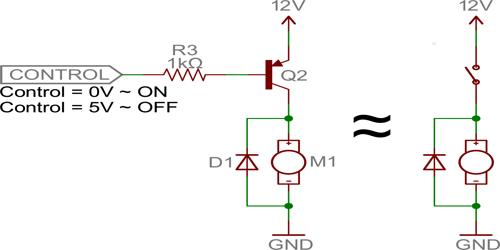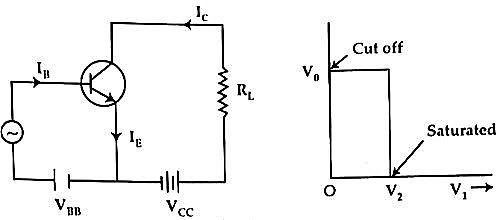Physics

# Use of Transistor as switchUse of Transistor as a switch

A transistor is a device that regulates current or voltage flow and acts as a switch or gate for electronic signals. It is composed of semiconductor material usually with at least three terminals for connection to an external circuit. It can act as a switch and an amplifier. It converts audio waves into electronic waves and resistor, controlling electronic current.

A transistor can be used as a switch. Transistor work as a switch when it is in cutoff and saturation regions. In this article, we will discuss how to use a transistor as a switch. In fig. (a) a ‘n-p-n’ transistor has been shown. It works as a switching circuit.

Working principle: In the figure, Vi = input voltage, V0 = output voltage = VCE =  potential difference between collector and emitter.

(1) When Vi = 0, then in the base-emitter junction there is no voltage i.e.. VBE = 0. So, base current IB = 0. Since IB = 0, so IC = 0. From figure (a) we get,

V0 = VS – ICRL.

Now since, IC = 0, when Vi = 0

So, V0 = VS, VS = supply voltage.

When Vi is increased slowly and until Vi > V1 i.e., Vi ≤ V1, then base current IB increases very little, IC also increases slightly. In this situations the transistor is said to be cut-off, then IC = 0.

Now if Vi increases and becomes V2 then collector current increases significantly and at that condition, the potential drop on RL is almost equal to the supply voltage VS. According to an equation, then VO = 0. If the input voltage is greater than V2, then, there does not occur a significant change in IC, so no influence on output voltage V0 is found. In this situation, the transistor is said to be saturated.So, it is seen that by changing input voltage the transistor can be changed into two states. One is cut off state (V0 + VS) and the other one is the saturated state (V0 = 0). The off and on a state of the transistor is used in a digital computer. A transistor can change state many hundred thousand times in a second. The output of a switch can be used as the input of the other switch and by connecting many switches together mathematical counting can be executed very rapidly.